# Introduction

This package implements a Bayesian changepoint detection algorithm of the flavor found in Stephens (1994). This approach assumes that given an average of several 2D crosscuts from a 3D bullet land scan which has had the global structure removed via the robust LOESS procedure Cleveland (1979), the land engraved area (LEA) is fairly flat, and the groove engraved area (GEA) possesses an approximately linear structure with a nonzero slope. An example of this basic structure is given below.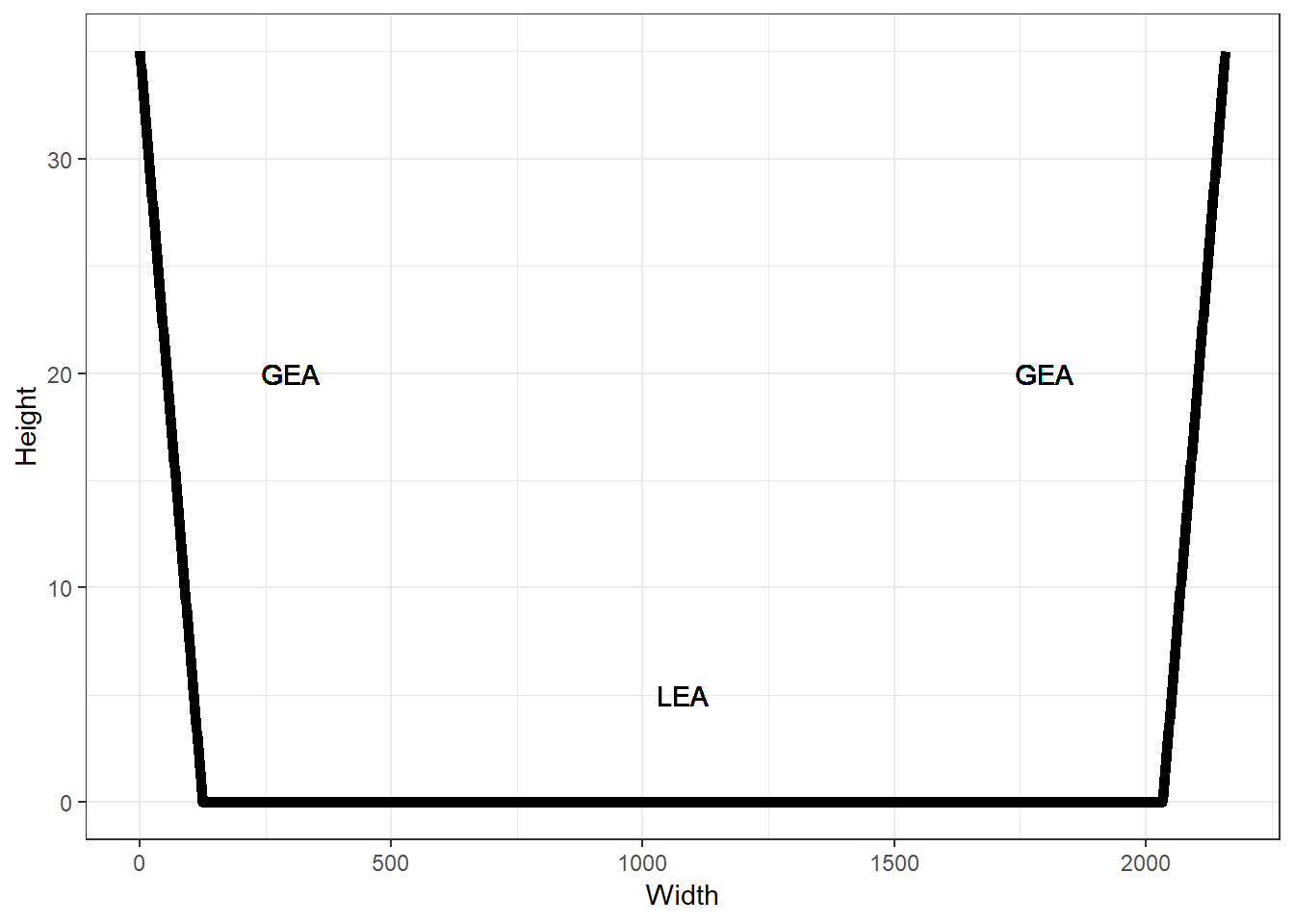The idea behind the changepoint approach is that within either the left GEA, right GEA, or the LEA, the global structure is consistent and can either be described by a line with zero slope, a line with positive slope for the right GEA, or a line with negative slope for the left GEA. Finding the points where the GEAs and LEA meet is treated as a problem of model selection. That is, the best fitting statistical model, in terms of the magnitude of the likelihood, should be the one which assumes that the points at which the global structure changes align with where the GEAs and LEA meet. These points of global structural change are what we will call changepoints. Thus, our model will be defined in a piecewise fashion. In practice there are also complex additional patterns which may exist for a number of reasons, but this large scale structural assumption remains generally reasonable. The complex smaller scale patterns can be thought of as the dependence in the data after accounting for the global structure. Because of the nature of the model which we consider, it becomes necessary for computational reasons to perform a couple of data preprocessing steps. Specifically, we will scale the residuals from the robust LOESS procedure, and we will impute missing values. In the next section, we describe the model that we will use to identify changepoints, after which we will describe the estimation procedure which we use. Following that, we will describe the two main data preprocessing steps necessary for estimation to be computationally feasible.

# Model

Before introducing the model, we introduce some notation. First, let $$\{Y(x_i): i = 1,2, ..., n\}$$ denote the set of random variables representing the residuals from the robust LOESS procedure at the values $$x_i$$. For simplicity, also assume that $$x_1 < x_2 < ... < x_n$$. Also, let $$c_l$$ be the value of the left changepoint and $$c_r$$ be the value of the right changepoint. Here, the left changepoint is where the left GEA meets the LEA, and the right changepoint is where the right GEA meets the LEA. Also, denote the median centered $$x$$ values as $$x'_i = x_i - \tilde{x}$$ where $$\tilde{x}$$ is the median $$x$$ value. As mentioned in the previous paragraph, the complex small scale patterns, such as the striae, will be modeled through a covariance structure on the data that will be allowed to differ between each GEA and between the GEAs and LEA. We will construct the covariance matrices from the exponential covariance function $$K(t, t';\sigma, \ell) = \sigma^2 e^{-\frac{|t - t'|}{\ell}} = cov(Y(t), Y(t'))$$. The differences in covariance matrices for the GEAs and LEA will be reflected in the parameters $$\sigma$$ and $$\ell$$. The data model that we consider is then,

\begin{align} (Y(x_1), Y(x_2), ..., Y(x_{k_1})) &\sim N(\beta_{01}\mathbb{1} + \beta_{11} x'_{1:k_1}, \Sigma_1(\sigma_1, \ell_1)) \\ (Y(x_{k_1 + 1}), Y(x_{k_1 + 2}), ..., Y(x_{k_2})) &\sim N(0, \Sigma_2(\sigma_2, \ell_2)) \\ (Y(x_{k_2 + 1}), Y(x_{k_2 + 2}), ..., Y(x_n)) &\sim N(\beta_{02}\mathbb{1} + \beta_{12} x'_{k_2 + 1:n}, \Sigma_3(\sigma_3, \ell_3)), \end{align}

where $$x_{k_1} < c_l \leq x_{k_1 + 1}$$ and $$x_{k_2} < c_r \leq x_{k_2 + 1}$$ Here, $$x_{1:k}$$ denotes the column vector $$(x_1, x_2, ..., x_k)^\top$$, and $$\mathbb{1}$$ denotes the vector of ones. Independence is assumed between each of these three distributions for simplicity.

Thus the parameters that need to be estimated include the four mean parameters in the GEAs, the six covariance parameters (two for each of the three areas), and the two changepoint parameters, $$c_l$$ and $$c_r$$.

The above model encapsulates the essence of the approach. However, there are a few difficulties. The first difficulty is that there are not always two GEAs in a particular land. There may be one GEA, or the land may only consist of the LEA. Thus, the above model is actually conditional on there being two GEAs in the data. We also define models for when there is one GEA on the left, one GEA on the right, or no GEAs. The models are defined in an essentially identical way. Conditional on there being only one GEA, the left GEA model is defined as,

\begin{align} (Y(x_1), Y(x_2), ..., Y(x_{k})) &\sim N(\beta_{0}\mathbb{1} + \beta_{1} x'_{1:k}, \Sigma_1(\sigma_1, \ell_1)) \\ (Y(x_{k + 1}), Y(x_{k + 2}), ..., Y(x_{n})) &\sim N(0, \Sigma_2(\sigma_2, \ell_2)), \end{align}

and the right GEA model is defined as,

\begin{align} (Y(x_{1}), Y(x_{2}), ..., Y(x_{k})) &\sim N(0, \Sigma_1(\sigma_1, \ell_1)) \\ (Y(x_{k + 1}), Y(x_{k + 2}), ..., Y(x_n)) &\sim N(\beta_{0}\mathbb{1} + \beta_{1} x'_{k + 1:n} \Sigma_2(\sigma_2, \ell_2)). \end{align}

Finally, conditional on there being no GEAs in the data, the model is simply

\begin{align} (Y(x_{1}), Y(x_{2}), ..., Y(x_{n})) &\sim N(0, \Sigma(\sigma, \ell)). \end{align}

Thus, estimating the changepoint locations also involves selecting the most appropriate model. In order to avoid confusion, we have slightly abused notation and, for example, $$\Sigma_1(\sigma_1, \ell_1)$$ as it is estimated in the two changepoint model is not the same as $$\Sigma_1(\sigma_1, \ell_1)$$ from either of the one changepoint models, and $$\Sigma_1(\sigma_1, \ell_1)$$ is also not the same between the two one changepoint models. As another example, $$\beta_0$$ is not the same between each of the one changepoint models. So, to be clear, duplication of notation in different models is not meant to imply that those parameters are shared between models.

Ultimately, these above four models are each individually fitted, and each model above is given a prior. From there, we do model selection in the formal Bayesian way, selecting the most probable model. Simultaneously with selecting the most probable model, we also use the maximum a posteriori estimator for the changepoint locations.

In order to complete a Bayesian model specification, we need priors on each of the parameters in each model as well as each model. We will assume independence between each parameter a priori. For each length scale $$\ell$$, we will assume $$\ell \sim \text{Gamma}(3,5)$$. For each standard deviation, we will assume $$\sigma \sim \text{Half-Normal}^{+}(0,1)$$, where $$\text{Half-Normal}^{+}(\cdot,\cdot)$$ is notation for the normal distribution restricted to the positive real numbers. For intercept parameters, $$\beta_{01}, \beta_{02}, \beta_0 \sim N(0, 10)$$. For the slope parameters, the preceding trend deviates slightly. For any slope that corresponds to the left GEA, $$\beta_1$$ or $$\beta_{01}$$, we will assume that the slope can not be positive. That is, $$\beta_1, \beta_{01} \sim \text{Half-Normal}^{-}(0,10)$$, where $$\text{Half-Normal}^{-}(\cdot, \cdot)$$ is notation for the normal distribution restricted to the negative real numbers. Contrastingly, for any slope that corresponds to the right GEA, $$\beta_1$$ or $$\beta_{02}$$, we will assume that the slope can not be negative. That is, $$\beta_1, \beta_{01} \sim \text{Half-Normal}^{+}(0,10)$$. For the changepoint locations, we assume a uniform prior $$\pi(c_l, c_r) \propto I(a < c_l < c_r - \gamma < b - \gamma)$$. Here, $$a$$ and $$b$$ are some values close to the edges of the data. How close those values are to the edges is a parameter that is set manually. Further, we include another hyperparameter, $$\gamma$$, which can be set so that the changepoints are not allowed to be too close to each other. This is also a parameter that is set manually. Lastly, we assume a uniform prior over all four models.

# Estimation

As was noted in Stephens (1994), for any model including a changepoint, the likelihood is not a smooth function of the changepoint location. This is because, holding all other parameters fixed, shifting the changepoint value will result in zero change to the likelihood until it crosses the nearest point to the right or left, at which point the likelihood makes a jump. This makes maximum likelihood estimation in the standard way infeasible, but Bayesian estimation can be done in a fairly straightforward way via Gibbs sampling. The basic idea is that, for each model, we can construct a two step Gibbs sampler. In step 1 we sample from the posterior distribution of the mean and covariance parameters given the changepoint locations, and in step 2 we sample from the changepoint locations given the mean and covariance parameters. Because of the non-conjugacy in our model, we perform both sampling steps using a random walk Metropolis-Hastings (RWMH) step with Gaussian proposals. For details on Gibbs sampling and the Metropolis-Hastings algorithm see Gelman et al. (2013). It is also worth mentioning that the zero changepoint model does not require Gibbs sampling at all, and we perform estimation there using a RWMH algorithm.

As a practical note, it turns out that the posterior distribution is almost always multimodal, and it can happen that the sampler gets stuck in a suboptimal mode for a large number of iterations. It is also the case that the suboptimal modes need not even be close to the groove locations. It has, however, been our experience that the optimal mode corresponds well to the actual groove locations, which are often somewhat close to the edges of the data. With this in mind, starting values and the RWMH proposal variances play a very important role in the success of the sampling algorithm. Fortunately, it seems to be the case that by setting the initial changepoint values close to the edges of the data and making the proposal variance small (around 100 seems to work well) allows the sampler to wander inwards, and even with a modest number of iterations (say 5000), typically pass through the largest mode corresponding to the groove locations. This is not always the case, and it is possible that increasing the number of iterations produces better results.

The sampling functions were originally written with the intention of tuning the proposal variances to potentially accelerate convergence, and thus several warmup iterations are required for this purpose. This turns out to be a bad idea in this context for two reasons. The first reason is that the warmup iterations allow the sampler to wander past the global modes and get stuck in suboptimal modes far from the groove locations, from which the sampler may or may not find its way back to the optimal modes in just a few thousand iterations. Secondly, if the sampler does wander past the optimal modes, which are usually on the edges of the data, the tuned proposal variance can be quite large. The large proposal variance might not be a huge problem if it weren’t for the fact that the width of the modes are almost always quite small. This means that it can take a very, very long time for the sampler to move from a suboptimal mode to the global mode. In order to mitigate this problem, we are currently setting the number of warmup iterations to be relatively small (somewhere in 100 to 500 seems to work well). In future iterations of these functions, we will remove the necessity of having warmup iterations or tuned proposal variances.

We now provide the two basic steps of the Gibbs sampler for the two changepoint case. The algorithms to sample from the other three models are omitted, and are nearly identical except for the a smaller number of parameters need to be sampled. Denote collection of mean and covariance parameters for the left GEA as $$\theta_1$$, the LEA as $$\theta_2$$, and the right GEA as $$\theta_3$$. Then, at iteration $$t$$ after warmup

1. given changepoint locations $$(c_l^{(t - 1)}, c_r^{(t - 1)})$$, sample $$(\theta_1^{(t)}, \theta_2^{(t)}, \theta_3^{(t)})$$ using independent RWMH steps for each $$\theta_i$$
2. given $$(\theta_1^{(t)}, \theta_2^{(t)}, \theta_3^{(t)})$$, sample $$(c_l^{(t)}, c_r^{(t)})$$ using a single RWMH step.

Initially, the Metropolis proposal variance for each $$\theta_i$$ is diagonal with diagonal elements all equal to $$1/2$$. The proposal variance for $$(c_l, c_r)$$ is initially set to be diagonal with elements equal to $$10^2$$. Note that because of the currently necessary warmup iterations, the variances after warmup for each $$\theta_i$$ becomes $$\frac{2.4^2}{d}\hat{Var}(\theta_i^{(1:w)}) + \text{diag}(0.1)$$, where $$d$$ is the dimension of $$\theta_i$$ (which is not constant between GEAs and LEA), and $$\hat{Var}(\theta_i^{(1:w)})$$ is the estimated variance covariance matrix from the $$w$$ warmup iterations. Note that the addition of a diagonal matrix with entries $$0.1$$ is to avoid the case when most or all warmup iterations have the same value. Similarly, the proposal variance for $$(c_l, c_r)$$ after warmup becomes $$\frac{2.4^2}{2}\hat{Var}((c_l,c_r)^{(1:w)}) + \text{diag}(1)$$.

After running the MCMC for each model, parameter estimates and the most likely model are jointly chosen according to the largest joint posterior value. That is, we arrive at estimates $$(\hat{\theta}, \hat{M}) = \underset{(\theta, M)}{\operatorname{argmax}}{\log(p(\theta, M | Y))}$$, where $$M$$ is the random variable associated with the choice of model, $$\theta$$ is the associated parameter vector for the appropriate model, and $$Y$$ is all of the available data.

# Data Preprocessing

Before running the analysis described above, we first perform two data preprocessing steps. The first step is to scale the residuals from the robust loess procedure by the standard deviation calculated from the entire set of residuals. The reason for this is simply to make priors for standard deviation and slope parameters easier to specify. For example, ensuring that the residuals are scaled to have standard deviation one means that the standard deviation parameters in our model should also be close to one. This scaling also ensures that slopes values are not very large.

The second preprocessing step is a bit more involved. In order to enable the algorithm to run reasonably fast, we need to take advantage of the sparse precision matrix structure that is induced by the covariance function we chose. Indeed, this was the reason for choosing this covariance function in the first place. Unfortunately, it is challenging to do this unless the observations are evenly spaced in the domain. In our case, this would be true if there were no missing values. In order to remedy this problem, we impute the missing data, but only in the case that there exist nonmissing observations outside of the missing values. In the case that the missing values exist on the edges of the data, we simply do not consider those domain values in the model.

We perform the imputation by treating the observations as coming from an unknown function, and infer the missing values from the known function values. In order to do this, we model the data with a Gaussian process and the squared exponential covariance function. That is, we suppose that

$Y(t) \sim \mathcal{GP}(0, K(t,t';\sigma^2, \ell)),$

where now $$K(t,t';\sigma^2, \ell) = \sigma^2 e^{-(t - t')^2/(2\ell^2)}$$ is the squared exponential covariance function. We emphasize for clarity that this is a different covariance function than we use in the changepoint model. The main reason for this is that in imputing values, it seems desirable to allow dependencies beyond immediately neighboring points to influence predictions as the function that we are trying to predict generally has a smooth global structure.

In the changepoint algorithm, we do allow the user to choose to estimate the Gaussian process parameters via maximum likelihood, which just uses the optim() function. However, we do not use the full set of data to estimate the parameters, as there is still too much to do estimation reasonably quickly. For this reason, we take a subset of the data chosen by selecting every 20-th point starting at the first observed location. This still provides plenty of data to estimate the covariance parameters well. However, we also supply default covariance parameter values so that estimation of these parameters is not necessary. These values were estimated from a representative bullet land, and we suspect that they will almost always work sufficiently well in practice.

When we impute the missing values, we use the estimated or default covariance parameter values and all of the observed data and compute the conditional mean of the missing values. To be clear, denote the distribution of the observed and missing data as

$(Y,Y^*)^\top \sim N\left( \begin{bmatrix} 0 \\ 0 \end{bmatrix}, \begin{bmatrix} \Sigma_{yy} & \Sigma_{yy^*} \\ \Sigma_{y^*y} & \Sigma_{y^*y^*}\end{bmatrix} \right).$

Here, $$Y$$ is observed data and $$Y^*$$ is the missing data, and the covariance matrix above is constructed from the squared exponential covariance function and the estimated or default covariance parameter values. We then use normal distribution theory to calculate the imputed values

$E(Y^*|Y = y) = \Sigma_{y^*y} \Sigma_{yy}^{-1}y$.

# Example

In this section, we will go through an example by individually using each necessary function as well as by using the wrapper function designed to perform all of the necessary steps with one function call. Data from this example comes from the the James Hamby study (Hamby, Brundage, and Thorpe 2009). We downsample the data to make the example run faster, but this would not be preferred in practice.

First, we read in the data. These data are an example of an average of several 2D cross cuts from a 3D bullet land scan in the Hamby 44 data set. We can tell both from the plot and the head(raw_data) call that there are missing values in the data.

library(bulletcp)
#>
#> Attaching package: 'dplyr'
#> The following objects are masked from 'package:stats':
#>
#>     filter, lag
#> The following objects are masked from 'package:base':
#>
#>     intersect, setdiff, setequal, union

data("example_data")
#>       x value
#> 1 0.000    NA
#> 2 0.645    NA
#> 3 1.290    NA
#> 4 1.935    NA
#> 5 2.580    NA
#> 6 3.225    NA
raw_data <- raw_data[seq(from = 1, to = nrow(raw_data), by = 30),]

ggplot(data = raw_data) +
geom_point(aes(x = x, y = value)) +
theme_bw() +
ylab("Height") +
xlab("Width")
#> Warning: Removed 11 rows containing missing values (geom_point).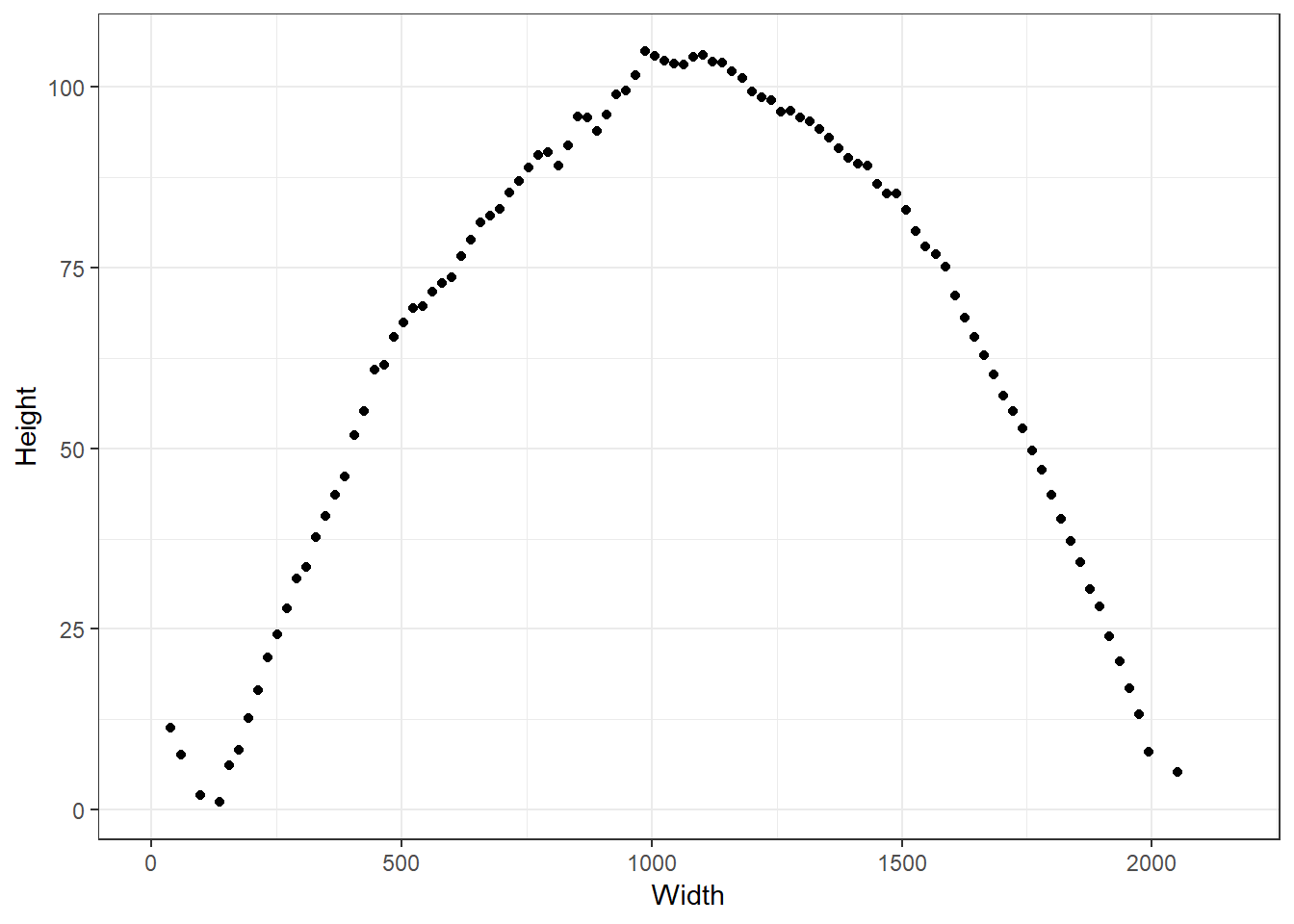A plot of an averaged cross cut from a 3D bullet land scan.

## Removing global structure

The data shown above still contains the global bullet curvature. The changepoint algorithm requires removal of this global structure before imputing the missing data. Functionality to do this is provided in the bulletxtrctr package (Hofmann, Vanderplas, and Krishnan 2018). Code to remove this structure is shown below.


# set the minimum y-value to zero
check_min <- min(raw_data$value[!is.na(raw_data$value)])
raw_data <- dplyr::mutate(raw_data, value_std = value - check_min)

# remove global structure
rlo_fit <- robust_loess_fit(cc = raw_data, iter = 20)
raw_data$rlo_pred <- predict(rlo_fit, newdata = raw_data) raw_data$rlo_resid <- raw_data$value_std - raw_data$rlo_pred

# define new data frame without the global structure
data <- data.frame("x" = raw_data$x, "y" = raw_data$rlo_resid)

# plot the new data
ggplot(data = data) +
geom_point(aes(x = x, y = y)) +
theme_bw() +
ylab("Height") +
xlab("Width")
#> Warning: Removed 11 rows containing missing values (geom_point).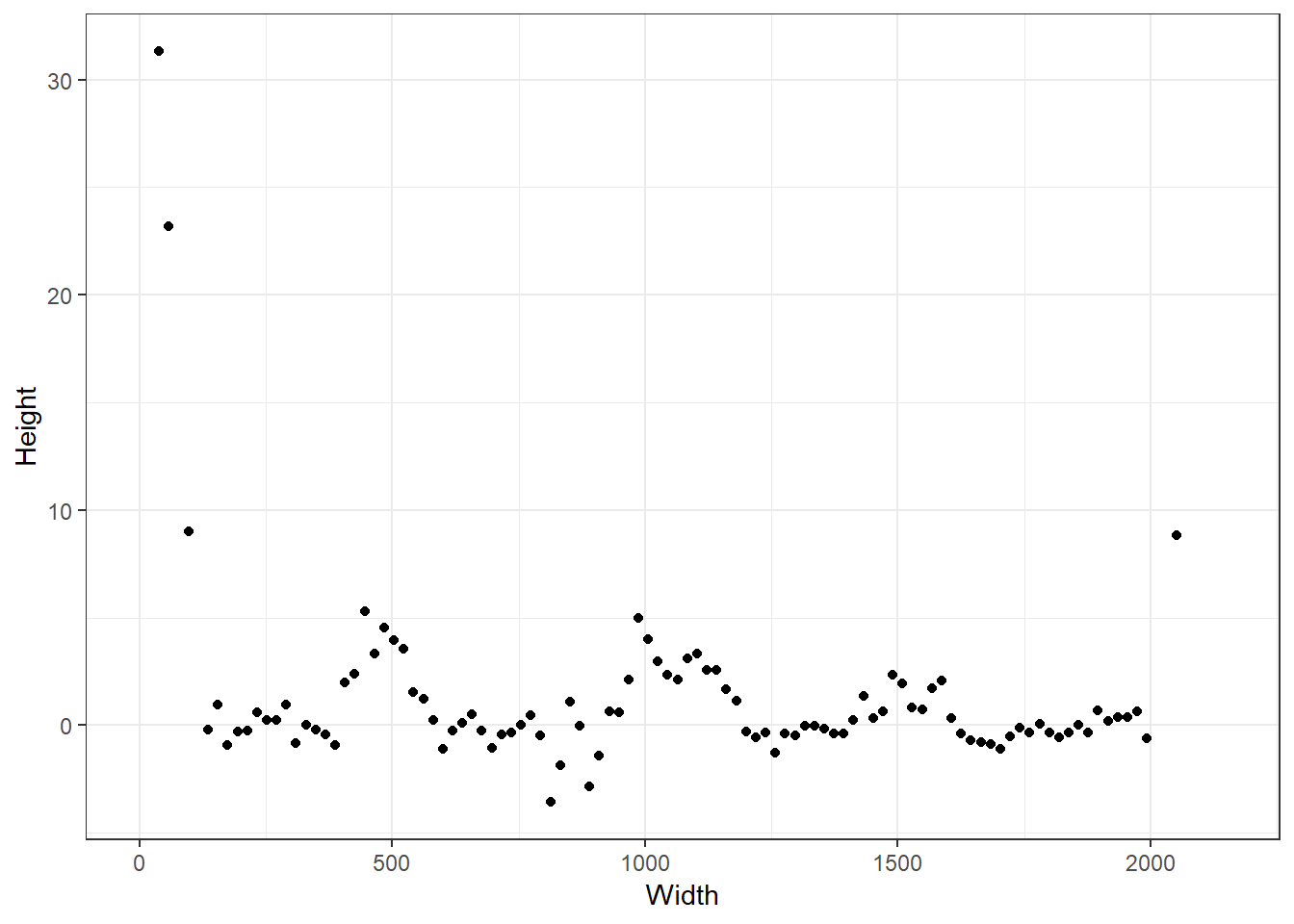## Imputing missing values

Now that the global structure has been removed from the land, we can proceed to impute missing values. We will work with scaled y-values, and so the first step is to perform this scaling.

# make range of x data equal to the range of non NA points and scale the y-values
d <- data.frame("x" = data$x, "y" = scale(data$y))
max_x_notNA <- max(d$x[!is.na(d$y)])
min_x_notNA <- min(d$x[!is.na(d$y)])
d <- d[d$x >= min_x_notNA & d$x <= max_x_notNA,]

Once the data have been scaled, we take a subset of them by selecting every 20-th point to use when estimating the Gaussian process parameters. Then, we estimate these parameters using the mlgp() function in this package. The optimization routine is done on the log scale so as to remove boundary constraints for the Gaussian process parameters, which are both constrained to be positive. The first parameter value returned is the log of the process standard deviation, and the second returned parameters value is the log of the length scale. The default values used if one does not choose to estimate the Gaussian process parameters are $$0.8$$ and $$15$$ for the standard deviation and length scale, respectively.

# subset data
temp_d <- d[seq(from = 1, to = nrow(d),by = 1),]

# remove missing data
temp_d <- temp_d[complete.cases(temp_d),]

# estimate the MLE's
mles <- mlgp(y = temp_d$y, x = temp_d$x)

# name MLE's impute_par
impute_par <- exp(mles$par) impute_par #>  0.9671305 22.2714492 Now that we have parameter estimates, we can use the function myimpute() to impute missing values. full_data <- imputeGP(y = d$y, x = d$x, sigma = impute_par, l = impute_par) head(full_data) #> x y #> 1 38.70 7.107245403 #> 2 58.05 5.181357035 #> 3 77.40 1.568790923 #> 4 96.75 1.831877298 #> 5 116.10 -0.000138855 #> 6 135.45 -0.337581864 ggplot(data = full_data) + geom_point(aes(x = x, y = y)) + theme_bw()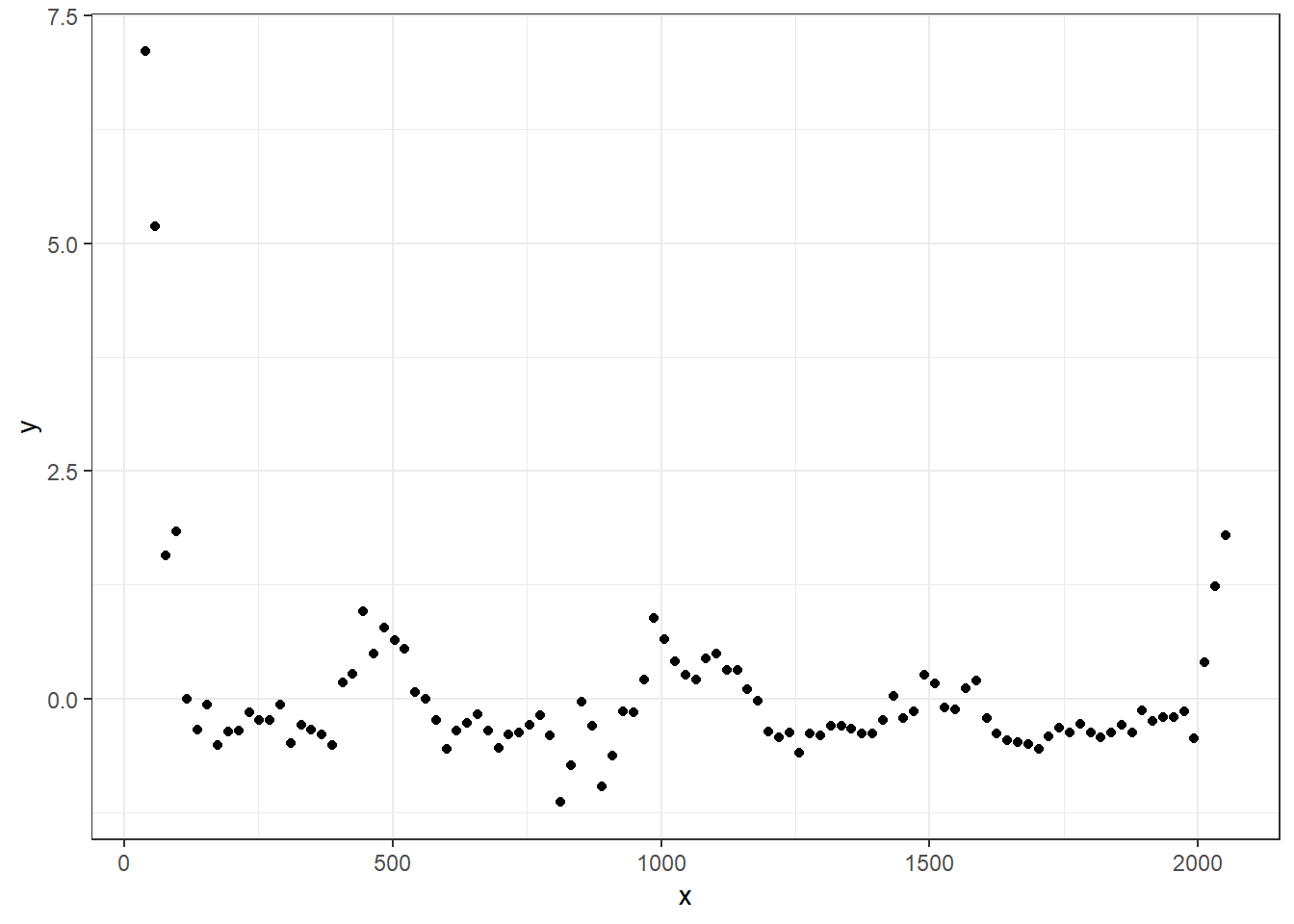## Estimating changepoint locations Now that we have imputed missing values, we can proceed to estimate the number and location of any grooves. In order to do this, we can can either call the function variable_cp_gibbs_v2(), which, in turn, calls functions for running separate Gibbs sampling algorithms in the 0, 1, or 2 changepoint cases, or we can call each of these functions individually. To clearly demonstrate what is going on, we will call each of the necessary functions directly. The only things that we really care about are the estimated groove locations, as well as the maximum value of the log posterior density (which occurs at the estimated groove locations). The latter will enable us to choose the number and location of any possible grooves while the former actually provides the estimated groove locations in each model. ### Model with no GEAs First, we will run a Gibbs sampler estimating model parameters in the case that there are zero changepoints. In this case, there should be no GEAs in the data. This is clearly not true in our example, but in some cases it will be. The function which runs the MCMC sampler in this case is named cp0_gibbs(). It may be worth noting that, although the function name would seem to imply that the algorithm is a Gibbs sampler, in the case of zero changepoints, it is actually just a RWMH algorithm. The function was named for the sake of consistency across sampling functions, which are always Gibbs samplers except in this special case. Argument descriptions for this function can be found in the relevant function documentation. In the beginning of the following code chunk, a function named lognormal_ou_pdf() is defined. This function defined the log of the pdf of a normal distribution with the covariance structure in the models described above. This function is necessary as it avoids matrix operations, taking advantage of the particular covariance structure we have defined. It is not critical to understand this function beyond the fact that it is needed in every MCMC sampler we employ. # log pdf of a normal distribution with the type of covariance matrix described in the model lognormal_ou_pdf <- function(x, mu, sigma, l) { n <- length(x) rho <- exp(-1/l) return(-n/2 * log(2 * pi) - n * log(sigma) - ((n - 1)/2) * log(1 - rho^2) - 1/2 * 1/(sigma^2 * (1 - rho^2)) * ((x - mu)^2 + (x[n] - mu[n])^2 + (1 + rho^2) * sum((x[2:(n-1)] - mu[2:(n-1)])^2) - 2 * rho * sum((x[1:(n-1)] - mu[1:(n-1)]) * (x[2:n] - mu[2:n])))) } # define starting values start.vals <- list("sigma" = c(1), "l" = c(10)) # proposal variance for the MH step prop_var <- diag(c(1/2,1/2)) # run the RWMH sampler for the zero changepoint model set.seed(1111) m0cp <- runmcmc_cp0(data = full_data, iter = 1000, start.vals = start.vals, prop_var = prop_var, warmup = 500, verbose = FALSE) # print maximum value of the (conditional) log posterior up to the normalizing constant max(m0cp$lpost)
#>  NA

### Model with one GEA

Now we proceed to the slightly more involved cases where the model assumes that there are one or more changepoints in the data. If we assume that there is one changepoint, we must model the case when the GEA is on the left side of the land as well as on the right side of the land. The functions that we will use to do this are named runmcmc_cp1_left() and runmcmc_cp1_right(), corresponding to the models with the location of the the GEA assumed to be on the left and right side of the land, respectively. We must specify more arguments to these functions than we did in the previous case. These arguments mostly correspond to parameters for the proposal variances for sampling the parameters on both the GEA and the LEA, as well as starting values for each of these sets of parameters. However, we must also specify the tol_edge argument which forces the potential changepoint to be a minimum distance from the edge of the data.


# define starting values for the changepoints
cp_start_left <- min(full_data$x) + 60 cp_start_right <- max(full_data$x) - 60

# define list of starting values for both the left and right changepoint models
start.vals <- list("left" = list("sigma" = c(1,1),
"l" = c(10,10),
"cp" = c(cp_start_left),
"beta" = c(-1),
"intercept" = c(0)),
"right" = list("sigma" = c(1,1),
"l" = c(10,10),
"cp" = c(cp_start_right),
"beta" = c(1),
"intercept" = c(0)))

# list of starting values for each of the two MH steps (not sampling the changepoint) for both the left and right changepoint models
prop_var <- list("left" = list(diag(c(1/2,1/2,1/2,1/2)),
diag(c(1/2,1/2))),
"right" = list(diag(c(1/2,1/2)),
diag(c(1/2,1/2,1/2, 1/2))))

# define the proposal variance for the RWMH step sampling the changepoint
cp_prop_var <- 10^2

# run Gibbs MCMC for the left GEA model
set.seed(1111)
m1cp_left <- runmcmc_cp1_left(data = full_data, iter = 1000, warmup = 500,
start.vals = start.vals$left, prop_var = prop_var$left,
cp_prop_var = cp_prop_var,
verbose = FALSE, tol_edge = 50)

# run Gibbs MCMC for the right GEA model
set.seed(1111)
m1cp_right <- runmcmc_cp1_right(data = full_data, iter = 1000, warmup = 500,
start.vals = start.vals$right, prop_var = prop_var$right,
cp_prop_var = cp_prop_var,
verbose = FALSE, tol_edge = 50)

# Find MAP estimates under both models of the changepoint location
map_cp1_left <- as.numeric(m1cp_left$parameters$cp)[which.max(m1cp_left$lpost)] map_cp1_right <- as.numeric(m1cp_right$parameters$cp)[which.max(m1cp_right$lpost)]

# print maximum value of (conditional) log posterior up to a normalizing constant
max(m1cp_left$lpost) #>  -39.2708 max(m1cp_right$lpost)
#>  -38.94021

# Plot the data and the changepoint MAPs
ggplot(data = full_data) +
geom_point(aes(x = x, y = y)) +
geom_vline(aes(xintercept = map_cp1_left)) +
geom_vline(aes(xintercept = map_cp1_right)) +
theme_bw()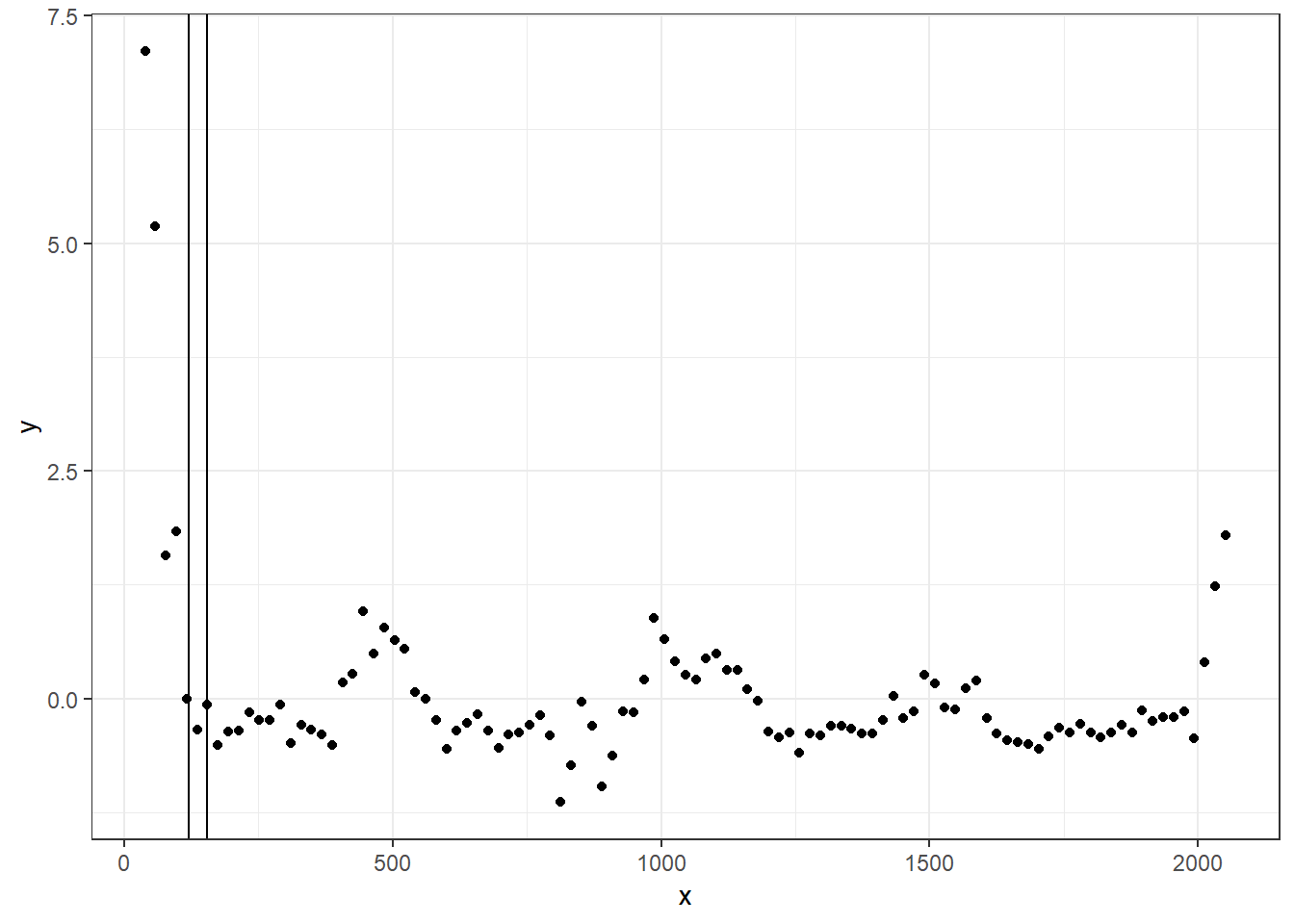In the plot above, the vertical black lines correspond to the MAP estimates of the changepoint locations for each model. Also, it is clear that the left changepoint model is a better fit to the data given the fact that the maximum log posterior value (up to a constant) is larger than it is for the right changepoint model. However, both of these models appear more likely than the zero changepoint model, which has a much lower maximum log posterior value.

Note that the function runmcmc_cp1() is just a wrapper for the runmcmc_cp1_left() and runmcmc_cp1_right() functions above. To use this function, starting values and proposal variances for each model are input separately. As shown below, the MAP estimates for the changepoints are very close to the estimates found from running each analysis individually as above. They will never be identical without setting a seed, as the MCMC algorithm is inherently random.


# run both Gibbs MCMC algorithms for each of the single changepoint model
set.seed(1111)
m1cp <- runmcmc_cp1(data = full_data, iter = 1000,
start.vals.left = start.vals$left, start.vals.right = start.vals$right,
prop_var_left = prop_var$left, prop_var_right = prop_var$right,
cp_prop_var = cp_prop_var,
tol_edge = 50,
warmup = 500, verbose = FALSE)

# Find MAP estimates under both models of the changepoint location
map_cp1_left2 <- as.numeric(m1cp$left_parameters$cp)[which.max(m1cp$lpost$left)]
map_cp1_right2 <- as.numeric(m1cp$right_parameters$cp)[which.max(m1cp$lpost$right)]

map_cp1_left2
#>  153.8055
map_cp1_right2
#>  165.0584

### Model with two GEAs

Because assuming a model structure with a single GEA involves fitting two models, fitting a model that assumes there are two GEAs is actually a bit simpler. Though it requires proposal variances and starting values for both GEAs as well as the LEA, it requires only performing one MCMC. The function that performs this sampling is named changepoint_gibbs_2cp_v2(), and its use is shown in the code block below. The one additional argument specific to this function is the named tol_cp. This is similar to the tol_edge argument except it specifies how close the changepoints can be to each other, not to the edge of the data. The default value for this argument is 1000, as this range is well below the range of the data.

# define starting values
start.vals <- list("sigma" = c(1,1,1),
"l" = c(10,10,10),
"cp" = c(cp_start_left, cp_start_right),
"beta" = c(-2,2),
"intercept" = c(0,0))

# define proposal variances (not for changepoints)
prop_var <- list(diag(c(1/2,1/2,1/2,1/2)),
diag(c(1/2,1/2)),
diag(c(1/2,1/2,1/2,1/2)))

# define proposal variance for changepoints
cp_prop_var <- diag(c(10^2, 10^2))

# run Gibbs MCMC
set.seed(1111)
m2cp <- runmcmc_cp2(data = full_data,
iter = 1000,
start.vals = start.vals,
prop_var = prop_var,
cp_prop_var = cp_prop_var,
tol_edge = 50,
tol_cp = 1000,
warmup = 500,
verbose = FALSE)

# Find MAP estimates of changepoint locations
map_cp2 <- m2cp$parameters$cp[which.max(m2cp$lpost),] # print maximum value of (conditional) log posterior up to a normalizing constant max(m2cp$lpost)
#>  -35.88717

# Plot the data and the changepoint MAPs
ggplot(data = full_data) +
geom_point(aes(x = x, y = y)) +
geom_vline(aes(xintercept = map_cp2)) +
geom_vline(aes(xintercept = map_cp2)) +
theme_bw()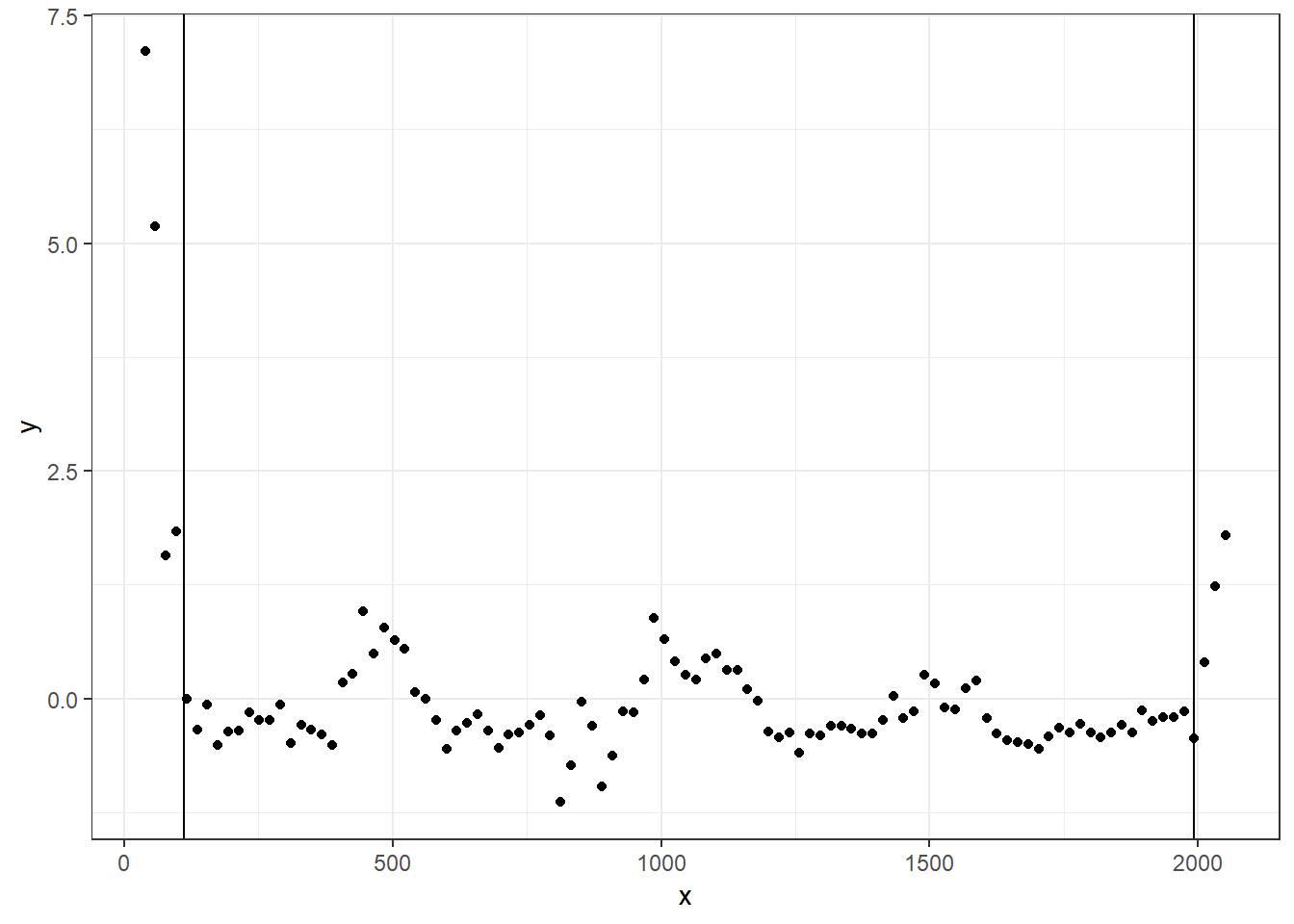This model has the highest maximum log posterior value, and thus will be chosen as the appropriate model for the data. Furthermore, the estimated changepoints appear to be very close to the true locations where the GEAs and the LEA meet.

### Running all MCMCs with one function

Of course, everything done above can be done much more simply by using one of the three wrapper functions designed to make this entire process simple. There are wrapper functions which differ only in terms of the preprocessing steps that they perform and the number of objects which they return. All of the functions run all of the MCMCs and estimate groove locations. We will now show how each of these functions is designed to be used in practice. The only additional argument that is required by these functions is the prior on the number of grooves named prior_numcp. This argument is a four element vector that must sum to 1. The elements of the prior vector are ordered as such (LEA only model, left GEA only model, right GEA only model, two GEA model). In practice it seems that the differences in the log posteriors (conditional on the model) are large enough that the model prior would need to be extraordinarily strong to affect the final results.

First, we will start with the function which does the least data preprocessing. This function is named runmcmc_cpall(). It requires that all data preprocessing be done beforehand, and it only runs the MCMCs and estimates the groove locations. We also need to specify lists of starting values and proposal variances. Each list element corresponds to a given model, so specification of starting values and proposal variances is a little cumbersome, but default starting values seem to work reasonably well on cases that have been tested so far. The list elements need to be named as they are in the example code below.


# changepoint starting values
cp_sval_left <- min(full_data$x) + 60 cp_sval_right <- max(full_data$x) - 60

# list of starting values for each model
start.vals <- list("cp2" = list("sigma" = c(1,1,1),
"l" = c(10,10,10),
"cp" = c(cp_sval_left,cp_sval_right),
"beta" = c(-2,2),
"intercept" = c(0,0)),
"cp1" = list("left" = list(
"sigma" = c(1,1),
"l" = c(10,10),
"cp" = c(cp_sval_left),
"beta" = c(-1),
"intercept" = c(0)),
"right" = list(
"sigma" = c(1,1),
"l" = c(10,10),
"cp" = c(cp_sval_right),
"beta" = c(1),
"intercept" = c(0))),
"cp0" = list("sigma" = c(1), "l" = c(10)))

# proposal variances for each model
prop_var <- list("cp2" = list(diag(c(1/2,1/2,1/2,1/2)),
diag(c(1/2,1/2)),
diag(c(1/2,1/2,1/2,1/2))),
"cp1" = list("left" = list(diag(c(1/2,1/2,1/2,1/2)),
diag(c(1/2,1/2))),
"right" = list(diag(c(1/2,1/2)),
diag(c(1/2,1/2,1/2, 1/2)))),
"cp0" = diag(c(1/2,1/2)))

# changepoint proposal variances
cp_prop_var <- list("cp2" = diag(c(10^2, 10^2)),
"cp1" = 10^2)

# prior on the number of changepoints
prior_numcp <- rep(1/4, times = 4)

set.seed(1111)
cp_gibbs <- runmcmc_cpall(data = full_data,
start.vals = start.vals,
prop_var = prop_var,
cp_prop_var = cp_prop_var,
verbose = FALSE,
tol_edge = 50,
tol_cp = 1000,
iter = 1000,
warmup = 500,
prior_numcp = prior_numcp)

# print the estimated maximum log posteriors
cp_gibbs$max_lpost #>$cp0
#>  NA
#>
#> $cp1_left #>  -40.48337 #> #>$cp1_right
#>  -40.69286
#>
#> $cp2 #>  -37.27347 # Estimated groove locations in each model cp_gibbs$cp_map
#> $2cp #>  110.4993 1993.0634 #> #>$1cp
#> $1cp$left
#>  148.1259
#>
#> $1cp$right
#>  127.2922

Now we introduce the usage of a function which, once global structure has been removed, will scale the data and impute missing values. The function is named detect_cp(). Notice that the object passed to the data argument is the unscaled data that still has missing values but has the global structure removed. Here, we have also set est_impute_par = TRUE indicating that we would like to estimate the imputation parameters. If this argument is set to FALSE, then the argument impute_par will need to be set with user supplied covariance parameters.


# Impute missing data, run MCMCs, and estimate MAPs
set.seed(1111)
cp_gibbs2 <- detect_cp(data = data,
start.vals = start.vals,
prop_var = prop_var,
cp_prop_var = cp_prop_var,
verbose = FALSE,
tol_edge = 50,
tol_cp = 1000,
iter = 1000,
warmup = 500,
prior_numcp = prior_numcp,
est_impute_par = TRUE)

# print the estimated log posteriors
cp_gibbs2$changepoint_results$max_lpost
#> $cp0 #>  NA #> #>$cp1_left
#>  -34.20846
#>
#> $cp1_right #>  -35.34848 #> #>$cp2
#>  -41.51201

# Estimated groove locations in each model
cp_gibbs2$changepoint_results$cp_map
#> $2cp #>  106.5513 1991.7210 #> #>$1cp
#> $1cp$left
#>  126.6153
#>
#> $1cp$right
#>  184.6226

# The groove locations automatically chosen based on the MAP criteria
cp_gibbs2$grooves #>  126.6153 2051.1000 Finally, we can call a function that also removes global structure, and thus can take in the raw crosscut data. This function is called get_grooves_bcp. The necessary arguments of this function follow a slightly different format than with the other functions so that it conforms to similar functions existing in the bulletxtrctr package. This function ultimately just removes the global structure and then calls detect_cp(). As such, the user can supply arguments to be passed to detect_cp, but it is intended that this not usually be necessary. The main reason why one may want to do this is to increase the number of iterations that each MCMC is run. The longer the MCMC is run, the more likely that the models can escape any local modes. For this reason, we demonstrate how a specific user supplied argument for the number of iterations can be fed to get_grooves_bcp. # Estimate the groove locations by supplying additional arguments cp_gibbs3 <- get_grooves_bcp(x = raw_data$x, value = raw_data$value, adjust = 10, iter = 1000) # Estimated groove locations cp_gibbs3$groove
#>   108.8436 2041.1000

# Acknowledgements

We would like to thank the efforts of CSAFE and the Roy J Carver High Resolution Microscopy lab in scanning the Hamby 44 set and providing the scans to us.